# Exponent Calculator

To calculate n raise to the power of x (xn), enter the value of base and exponent in the given input boxes and hit the "calculate" button of this exponent calculator## Exponent Calculator

Exponents Calculator or e calculator is used in solving exponential forms of expressions. It is also known as raised to the power calculator.

### Properties of exponents calculator:

This calculator solves bases with both negative exponents and positive exponents. It also provides a step by step method with an accurate answer.

## What is an exponent?

An exponent is a small number located in the upper, right-hand position of an exponential expression (base exponent), which indicates the power to which the base of the expression is raised.The exponent of a number shows you how many times the number is to be used in multiplication. Exponents do not have to be numbers or constants; they can be variables.

They are often positive whole numbers, but they can be negative numbers, fractional numbers, irrational numbers, or complex numbers. It is written as a small number to the right and above the base number.

Types:

There are basically two types of exponents.

• ### Positive exponent

A positive exponent tells how many times a number is needed to be multiplied by itself. Use our exponent calculator to solve your questions.

• ### Negative exponent

A negative exponent represents which fraction of the base, the solution is. To simplify exponents with power in the form of fractions, use our exponent calculator.

### Example:

Calculate the exponent for the 3 raised to the power of 4 (3 to the power of 4).

It means = 34

Solution:

3*3*3*3 = 81

4 to the 3rd power = 81

Therefore the exponent is 81

2 raised to the power calculator.

### Example:

What is the value of exponent for 2 raise to power 9 (2 to the 9th power)

It means = 29

Solution:

2*2*2*2*2*2*2*2*2 = 512

2 to the 9th power = 512

Therefore the exponent is 512.

### Example:

How do you calculate the exponents of 5,6,7 to the power of 4?

It means = 54, 64, 74

Solution:

5*5*5*5 = 625

6*6*6*6 = 1296

7*7*7*7 = 2401

Therefore the exponents are 625, 1296, 2401.

## How to calculate the nth power of a number?

The nth power of a base, let’s say “y”, means y multiplied to itself nth time. If we are to find the fifth power of y, it is y*y*y*y*y.

Some other solutions for the nth power calculator are in the following table.

 0.1 to the power of 3 0.001 0.5 to the power of 3 0.125 0.5 to the power of 4 0.0625 1.2 to the power of 4 2.0736 1.02 to the 10th power 1.21899 1.03 to the 10th power 1.34392 1.2 to the power of 5 2.48832 1.4 to the 10th power 28.9255 1.05 to the power of 5 1.27628 1.05 to the 10th power 1.62889 1.06 to the 10th power 1.79085 2 to the 3rd power 8 2 to the power of 3 8 2 raised to the power of 4 16 2 to the power of 6 64 2 to the power of 7 128 2 to the 9th power 512 2 to the tenth power 1024 2 to the 15th power 32768 2 to the 10th power 1024 2 to the power of 28 2.68435e+08 3 to the power of 2 9 3 to the 3 power 27 3 to the 4 power 81 3 to the 8th power 6561 3 to the 9th power 19683 3 to the 12th power 531441 3 to what power equals 81 34 4 to the power of 3 64 4 to the power of 4 256 4 to the power of 7 16384 7 to the power of 3 343 12 to the 2nd power 144 2.5 to the power of 3 15.625 12 to the power of 3 1728 10 exponent 3 1000 24 to the second power (242) 576 10 to the power of 3 1000 3 to the power of 5 243 6 to the power of 3 216 9 to the power of 3 729 9 to the power of 2 81 10 to the power of 5 100000

## Exponent Rules:

Learning the exponent rules along with log rules can make maths really easy for understanding. There are 7 exponent rules.

• ### Zero Property of exponent:

It means if the power of a base is zero then the value of the solution will be 1.Example: Simplify 50.

In this question, the power of base is zero, then according to the zero property of exponents, the answer of this non zero base is 1. Hence,

50= 1

• ### Negative Property of exponent:

It means when the power of base is a negative number, then after multiplying we will have to find the reciprocal of the answer.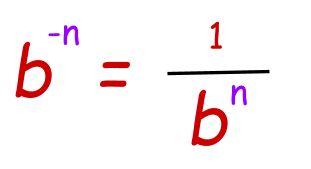Example: Simplify 1/3-2.

We will first make the power positive by taking reciprocal.

1/3-2=32

32 = 9

• ### Product Property of exponent:

When two exponential expressions having the same non zero base and different powers are multiplied, then their powers are added over the same base.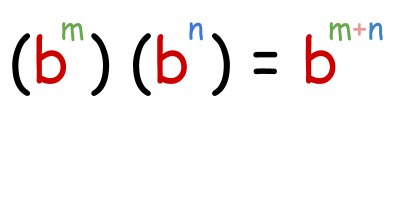Example: Solve (26)(22).

As it is obvious, bases are the same so powers are to be added. Now

(26)(22) = 26+2

28 =2*2*2*2*2*2*2*2

=256

• ### Quotient Property of exponent:

It is the opposite of the product property of exponent. When two same bases having different exponents are required to be divided, then their powers are subtracted.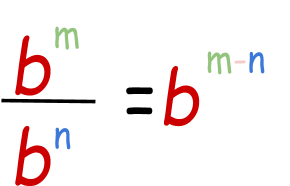Example: Simplify 37 /32

3/ 32=37-2

35=3*3*3*3*3

= 243

• ### Power of a Power Property:

When an exponent expression further has power, then firstly you need to multiply the powers and then solve the expression.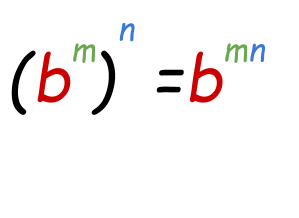Example: Solve: ( x2)3.

Keeping in view the power of power property of exponents, we will multiply powers.

(x2)3=x2*3

= x6

• ### Power of a product property:

When a product of bases is raised to some power, the bases will possess the power separately.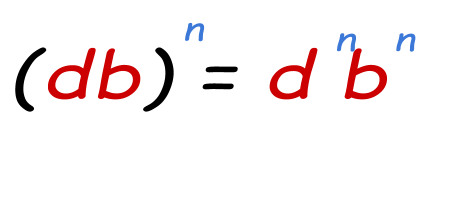Example: Simplify (4*5)2

452=16*25

= 400

• ### Power of a Quotient Property:

It is the same as the power of a product property. Power belongs separately to both the numerator and denominator.Example: Solve (2/3)2

(2/3)2=22 / 32

2/ 32=4/9

1. Mclph.umn.edu. 2020. What Is An Exponent?.
2. Stapel, E., 2020. Exponents: Basic Rules | Purplemath.
3. Math.com. 2020. Algebra Basics - Exponents - In Depth.
4. Mathinsight.org. 2020. Basic Rules For Exponentiation - Math Insight.
5. Analyzemath.com. 2020. What Are Exponents In Maths - Grade 7 Maths Questions With Detailed Solutions.